Home MonkeyNotes Printable Notes Digital Library Study Guides Study Smart Parents Tips College Planning Test Prep Fun Zone Help / FAQ How to Cite New Title Request

6.4 Substitution and Change of Variables

In this method, we transform the integral to a standard form by changing the variable x of the integration to t by means of a suitable substitution of the type x = f ( t ).

Theorem : If x = f ( t ) is a differentiable function of t,

Then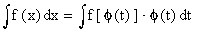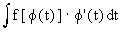Note : Comparing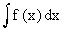with , we observe that dx is replaced

by. Hence supposing dx and dt as if they were separate entities, we have the following working rule :This technique is often compared with the chain - rule of derivatives since they both apply to composite functions.

Example 29

Evaluate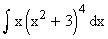Solution :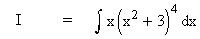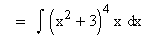Here the inner function of the composite function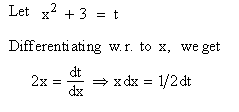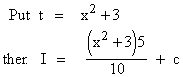Your browser does not support the IFRAME tag.

Example 30

EvaluateSolution : Let 4 - 3x = t,

then -3 = dtExample 31

EvaluateSolution : Let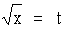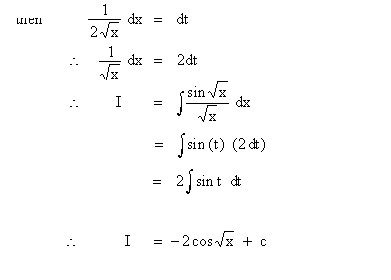Example 32

Evaluate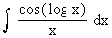Solution : Let log x = t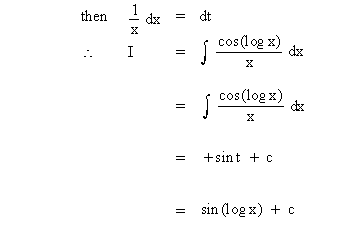Example 33

Evaluate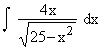Solution : Let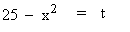then -2 x dx = dt

\ 2x dx = -dtExample 34

EvaluateSolution : Let arc tan x = tExample 35

Evaluate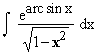Solution : Let arc sinx = t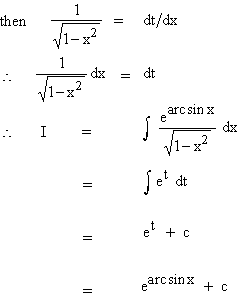Example 36

Evaluate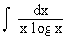Solution : Let log x = t

ThenIndex

6.1 Anti-derivatives (indefinite Integral)
6.2 Integration Of Some Trignometric Functions
6.3 Methods Of Integration
6.4 Substitution And Change Of Variables
6.5 Some Standard Substitutions
6.6 Integration By Parts
6.7 Integration By Partial Fractions

Chapter 7